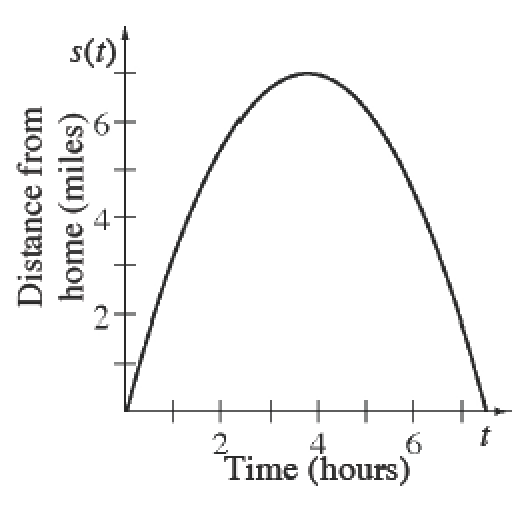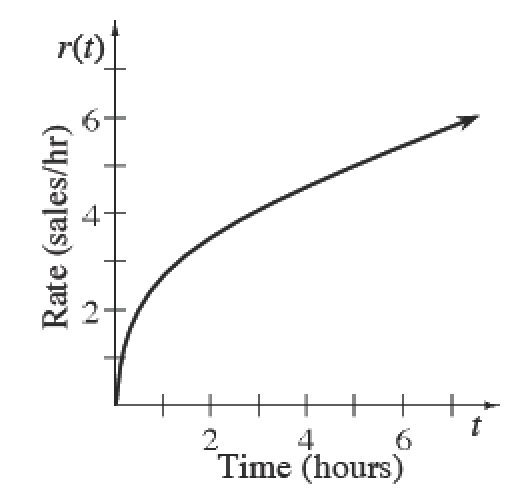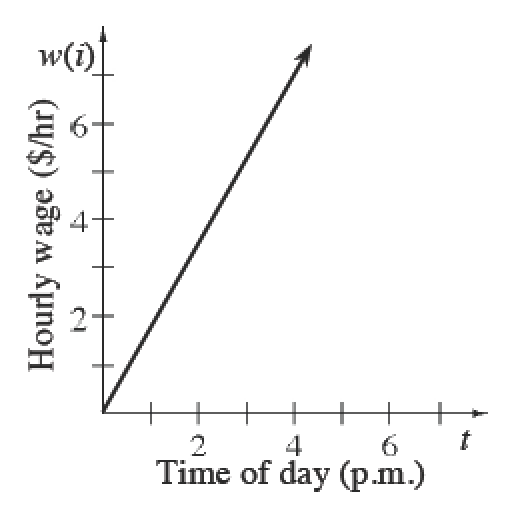### Home > APCALC > Chapter 4 > Lesson 4.3.1 > Problem4-110

4-110.

The three graphs below each tell a story. Examine each graph carefully and then complete each part below. Pay close attention to the labeling of the $x$- and $y$-axes.

1. The graph of $y = s\left(t\right)$ involves a bicycle. Use a complete sentence to describe the physical meaning of $s^\prime\left(6\right)$. Include units of measure.

$s'(t)=\frac{ds}{dt}=\text{ instantaneous }\frac{\Delta \text{feet}}{\Delta \text{time}}$1. The graph of $y = r\left(t\right)$ involves an employee of a calculator company. Use a complete sentence to $\int _ { 0 } ^ { 5 } r ( t ) d t$. Include units of measure.

$\frac{\text{sales}}{\text{hour}}$

indicates a rate graph and an integral is an area function.

Be sure to mention the significance of the bounds.1. The graph of $y = w\left(t\right)$ involves a babysitter. Use a complete sentence to describe the physical meaning of $\int _ { 4 } ^ { 10 } w ( i ) d i$. Include units of measure.

Refer to the hints in part (b).